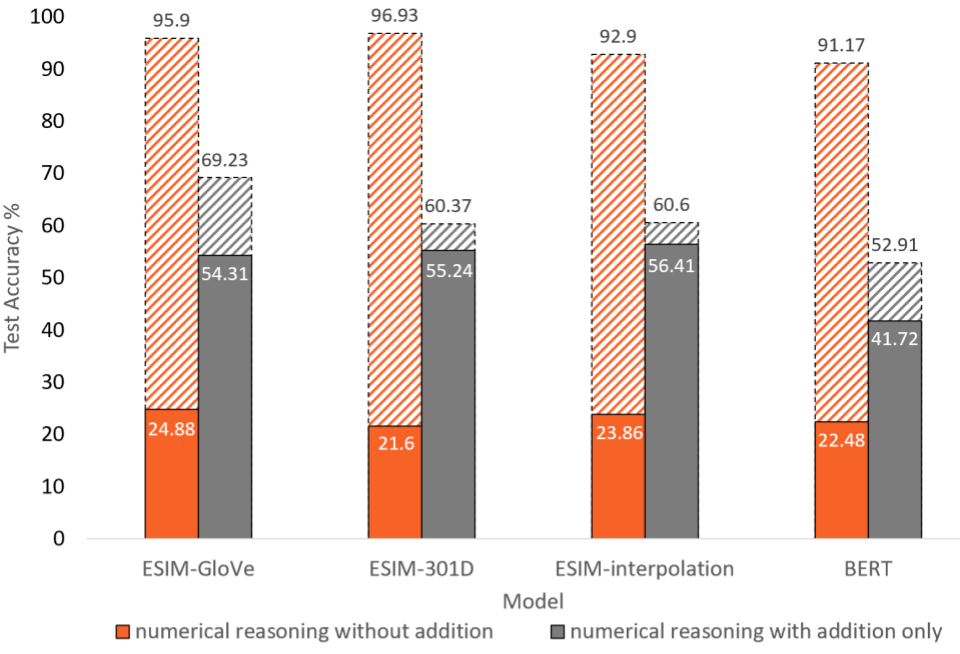# Breaking Numerical Reasoning in NLI

Are natural language inference (NLI) deep learning models capable of numerical reasoning? This is the question that led to my course project in “Natural Language Understanding and Computational Semantics” course at NYU Center for Data Science. In summary, my teammates and I tried adversarial data augmentation and modified numerical word embedding to show that some of SoTA NLI architectures at the time could not perform correct numerical reasoning that involve adding multiple number words.

Natural Language Inference is a classification task with sentence pairs. For each pair of English sentences, the model should classify the second sentence (hypothesis) as entailment (definitely true), contradiction (definitely false), or neutral (maybe true) given the first sentence (premise). For example, this sentence pair (‘A man holds two children in his arms.’, ‘Three humans together.’) should be entailment. You can find more about NLI task on this page.

We found that if we modify some sentence pairs by simply changing number words, then models make incorrect classifications. For example, when I change the aformentioned sentence pair to (‘A man holds two children in his arms.’, ‘Four humans together.’), ESIM model still predicts entailment even though the premise does not necessarily imply the hypothesis. It seems to imply that the model did not learn to use number words in classification at all.

This could mean one of the two things. First, it might mean that the dataset we used (SNLI) doesn’t contain enough examples where the numerical reasoning is a crucial factor of the prediction. If matching objects (e.g. ‘man’ and ‘human’) is enough to correctly predict most sentence pairs, then the model might never learn to pick up number words. Second, it might mean that the architecture itself is incapable of performing numerical reasoning. We hypothesized this because many NLI architectures were based on inter-sentence word-pair attention even though addition requires considering >= 3 words at once.

To find out which is the case, we augmented the data by changing number words and objects in the existing sentence pairs in SNLI. We augmented all 3 classes as follows:

• Entailment if both numbers and objects match
• Contradiction if the objects from the two sentences have antonym relationship
• e.g. “the two boys are swimming with boogie boards” (Premise) and “the two girls are swimming with their floats” (Hypothesis).
• Neutral if the numerical value in hypothesis is bigger than in premise for the matching object

The result is shown in the figure below.

1. Performance of SoTA NLI models break on our modified test sets.
2. Data augmentation in training improves the performance for the modified test set that requires numerical reasoning without addition.
• For example, training data augmentation in ESIM-GloVe improved the test performance from 24.88 to 95.9.
3. However, our data augmentation does not improve the performance for the modified test set that requires addition as much as (2).
• This might mean that these architectures cannot learn to perform complicated numerical reasoning beyond simple word-pair pattern matching.Our result. Data augmentation helps sentence pairs without addition but not with addition.

This project was chosen as one of the four exemplary projects in Spring 2019. If you are interested, you can access the full paper by selecting reasoning.pdf at this link.To find the pdf file, select ‘reasoning.pdf’ file from the link.##### Jungkyu Park
###### Deep Learning Researcher, PhD Student

Efficient Deep learning in medical imaging